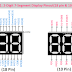Here, in this article, we are going to see the pinout diagram of a 2-digit, 3-digit, and 4-digit 7-segment Display. 2-Digit, 3-Digit, and 4-Digit 7-Segment Displays are also known as Multi-Digit Displays. They are types of numeric displays that consist of multiple 7-segment digits arranged together to show multi-digit numbers. These multi-digit displays are commonly used in various applications where numerical information needs to be shown.

## 2-Digit 7-Segment Display Pinout

A 2-digit 7-segment display consists of two separate sets of seven-segment 1-digit displays, side by side. Each set can display a single digit from 0 to 9. This type of display is often used in digital clocks, timers, counters, and devices where two-digit numbers need to be displayed simultaneously. Each digit can be controlled independently to show different numbers. In a 2-digit display, we can display values from 0 to 99.

Here, you can see the terminal identification and Pinout diagram of a 2-digit 7-segment Display.

There are two types of 2-digit Seven-Segment Displays available in the market - 18 Pin and 10 Pin. The 18 Pin display provides all the pins from the two individual 1-digit Seven-Segment Displays. Whereas the 10-pin Display uses a multiplexing system where segment pins are connected to each other so it provides the same segment pins for both displays and it provides two digit selector terminals. Using these digit terminals we can control both displays.

## 3-Digit 7-Segment Display Pinout

A 3-digit 7-segment display features three sets of 7-segment digits arranged side by side. Just like the 2-digit display, each digit can show numbers from 0 to 9. These displays are commonly used in applications where three-digit numbers need to be displayed, such as temperature readouts, digital meters, and countdown timers. In a 3-digit display, we can display values from 0 to 999.

Here, you can see the Pinout Diagram of a 3-digit 7-segment Display.

As you can see the 3-digit seven-segment display has a total of 12 Pins. Here, 8 of the pins are for the 8 LEDs on each of the 7 segment displays, which includes A-G and DP (decimal point). Pin no. 6 is not connected. The other 3 pins represent each of the 3 digits from DIG 1-DIG3.

## 4-Digit 7-Segment Display Pinout

A 4-digit 7-segment display comprises four sets of 7-segment digits placed side by side in a row. Each digit can display numbers from 0 to 9 independently. This type of display is widely used in digital clocks, scoreboards, electronic measurement instruments, and other devices that require the display of four-digit numbers. In a 4-digit display, we can display values from 0 to 9999.

Here, you can see the Pinout Diagram of a 4-digit 7-segment Display.

You can see here also, the 4-digit seven-segment display has a total of 12 Pins. Here, 8 of the pins are for the 8 LEDs on each of the 7 segment displays, which includes A-G and DP (decimal point). The other 4 pins represent each of the 4 digits from DIG1-DIG4.

Thank you for visiting the Website. Keep visiting for more Updates.

2-Digit, 3-Digit, and 4-Digit(12 Pin) 7-Segment Display PinoutReviewed by Author on September 12, 2023 Rating: 5